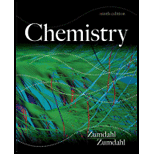# Consider the following statements: “Consider the reaction A ( g ) + B ( g ) ⇌ C ( g ) , for which at equilibrium [A] = 2 M, [B] = 1 M, and [C] = 4 M. To a 1-L container of the system at equilibrium, you add 3 moles of B. A possible equilibrium condition is [A] = 1 M, [B] = 3 M, and [C] = 6 M because in both cases K = 2.” Indicate everything that is correct in these statements and everything that is incorrect. Correct the incorrect statements, and explain.### Chemistry

9th Edition
Steven S. Zumdahl
Publisher: Cengage Learning
ISBN: 9781133611097### Chemistry

9th Edition
Steven S. Zumdahl
Publisher: Cengage Learning
ISBN: 9781133611097

#### Solutions

Chapter
Section
Chapter 13, Problem 7ALQ
Textbook Problem

## Expert Solution

### Want to see the full answer?

Check out a sample textbook solution.See solution

### Want to see this answer and more?

Experts are waiting 24/7 to provide step-by-step solutions in as fast as 30 minutes!*

See Solution

*Response times vary by subject and question complexity. Median response time is 34 minutes and may be longer for new subjects.